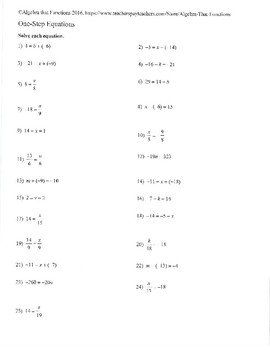Equations One-Step Practice/Review *DISTANCE LEARNING5th - 12th, Adult Education, Homeschool
Subjects
Standards
Resource Type
Formats Included
• PDF
•Activity
Pages
6 pages
Easel Activity Included
This resource includes a ready-to-use interactive activity students can complete on any device. Easel by TpT is free to use! Learn more.

Description

PRINT and DIGITAL * * Makes distance planning so much easier!

Equations One-Step Practice/Review

Equations One-Step Practice/Review Practice product has a total of 50 equations with no prep required by you! These one-step equations are perfect for giving your students the practice they need to master solving one-step equations. They are also great for sub days because there is no prep for you!

There are two levels of one-step equations included. Twenty-five of the one-step equations are integer equations and 25 are decimal equations. These one-step equations require the use of addition, subtraction, multiplication, and division. You can differentiate according to your students’ needs!

You can use these one-step equations in many ways. You can use them for, individual practice, partner practice, small group practice, whole class practice, warm-ups, games, review, diagnostic assessments, formative assessments, and summative assessments. My Equations One-Step Practice/Review product is a must-have product that will make your life so much easier and help your students master solving equations!

Algebra that Functions and it is for use in one classroom only. This product is also bound by copyright laws. Redistributing, editing, selling, or posting this item (or any part thereof) on the Internet are all strictly prohibited without first gaining permission from the author. Violations are subject to the penalties of the Digital Millennium Copyright Act. Please contact me if you wish to be granted special permissions!

This is a Great Companion Activity to my

Equations Color Math

Equations: Find, Fix, and Analyze Errors

Equations Enrichment

Equations With Fractions

Equations With Proportions

Multi-Step Equations

Multi-Step Equations With Infinite Solutions, No Solutions, and Integer Solutions

Equations Distributive Property with Fraction and Integer Coefficients Practice with Worked Out Solutions

Absolute Value Equations

Learning Goals and Scale

Identifying Critical Information

Revising Knowledge

Customer Tip!

You can get TPT credit to use on future purchases!

Please go to your My Purchases page (you may need to login). Beside each purchase you'll see a Provide Feedback button. Simply click it and you will be taken to a page where you can give a quick rating and leave a short comment for the product.

Each time you give feedback, TPT gives you feedback credits that you use to lower the cost of your future purchases. I value your feedback greatly as it helps me determine which products are most valuable for your classroom so I can create more for you.

Be the first to know about my new discounts, freebies and product launches! CLICK ON THE GREEN STAR next to my store logo to become a follower. You will receive updates about my store.

Total Pages
6 pages
Included
Teaching Duration
N/A
Report this Resource to TpT
Reported resources will be reviewed by our team. Report this resource to let us know if this resource violates TpT’s content guidelines.

Standards

to see state-specific standards (only available in the US).
Solve linear equations with rational number coefficients, including equations whose solutions require expanding expressions using the distributive property and collecting like terms.
Solve multi-step real-life and mathematical problems posed with positive and negative rational numbers in any form (whole numbers, fractions, and decimals), using tools strategically. Apply properties of operations to calculate with numbers in any form; convert between forms as appropriate; and assess the reasonableness of answers using mental computation and estimation strategies. For example: If a woman making \$25 an hour gets a 10% raise, she will make an additional 1/10 of her salary an hour, or \$2.50, for a new salary of \$27.50. If you want to place a towel bar 9 3/4 inches long in the center of a door that is 27 1/2 inches wide, you will need to place the bar about 9 inches from each edge; this estimate can be used as a check on the exact computation.
Solve real-world and mathematical problems by writing and solving equations of the form 𝘹 + 𝘱 = 𝘲 and 𝘱𝘹 = 𝘲 for cases in which 𝘱, 𝘲 and 𝘹 are all nonnegative rational numbers.
Use variables to represent numbers and write expressions when solving a real-world or mathematical problem; understand that a variable can represent an unknown number, or, depending on the purpose at hand, any number in a specified set.
Understand solving an equation or inequality as a process of answering a question: which values from a specified set, if any, make the equation or inequality true? Use substitution to determine whether a given number in a specified set makes an equation or inequality true.